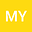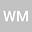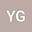A Barycentric Rational Interpolation Collocation Method for Solving the Helmholtz Equation
•••• Miaomiao Yang,
• Wentao Ma,
• Yongbin Ge
Miaomiao Yang
Ningxia University

Corresponding Author:yangmiao6163@163.com

Author ProfileWentao Ma
Ningxia University
Author ProfileYongbin Ge
Institute of Applied Mathematics and Mechanics
Author Profile## Abstract

In this paper, we developed a meshless collocation method by using barycentric rational interpolation basis function based on the Chebyshev to deduce the scheme for solving the Helmholtz equation defined in arbitrary domain with complex boundary shapes. Firstly, the spatial variables and their partial derivatives are treated by interpolation basis functions, and the collocation method for solving second order differential equations is established. Then the differential matrix is used to simplify the differential equations on a given test node. Finally, numerical experiments based on three kinds of test nodes show that the proposed method can be used to calculate not only the high wave numbers problems, but also the variable wave numbers problems. Moreover, the algorithm has the advantages of high calculation accuracy, good numerical stability and the less CPU time consuming.